Browse by Topic

# Lessons Learned Migrating from Python to the Wolfram LanguageSince I started working at Wolfram, I have been almost exclusively using Mathematica, not only as computing software but also as a program in which to write documents. I very quickly felt comfortable using Mathematica in both of these capacities, but I had yet to truly use it as a programming platform with the Wolfram Language.

I discovered Wolfram in high school, where I was—and still am!—fascinated by Wolfram|Alpha’s natural language capability and knowledgebase. This interest continued through college, where I was classically trained in both Python and Java but continued to use Wolfram|Alpha for math and chemistry. My undergraduate research demanded that I learn bash scripting and Tcl and that I continue using Python.

I was introduced to Mathematica in graduate school in my advanced quantum mechanics course, during the first semester of my Ph.D. The research scientist in my Ph.D. research group is a strong Mathematica user and suggested I double-check results originally analyzed using Python with his Mathematica notebook. Although I had been exposed to Mathematica multiple times during my Ph.D., I had never thought of the Wolfram Language being comparable to Python and didn’t realize it could be used for “actual” programming until I started working at Wolfram.

So one Saturday, I sat down at my computer to figure out the Wolfram Language’s functionality by rewriting an assignment from my undergraduate Introduction to Computing course. I could quickly mirror my undergrad Python code in the Wolfram Language, but ended up learning two very important lessons:

• The Wolfram Language works best when written to take advantage of its strengths instead of mirroring programming styles used by other languages.
• The Wolfram Language has advantages over other languages with its built-in access to dynamic, real-world data.

## My First Python Project

As an undergrad, my first project using Python was writing a Monte Carlo simulation that was based on a set of previous example returns to estimate a simple return on investment.

In this assignment, we had to:

• Write the Monte Carlo simulation in Python
• Import the output from our code into R
• Analyze our simulation in R
• Write a discussion on our simulation and the results

When redoing this project in the Wolfram Language, I decided to configure Python to run within my package of Mathematica. So I followed these instructions to configure Python in Mathematica. This process allowed me to take my code and evaluate it for comparable speeds.

Now, let me walk you through this assignment. One of the first steps is to assign a variable to the list of percentages of returns on investment between 2004 and 2014. This task is simple for both programming languages, but in the Wolfram Language, we can pull the actual return on investment data directly from the Wolfram Knowledgebase: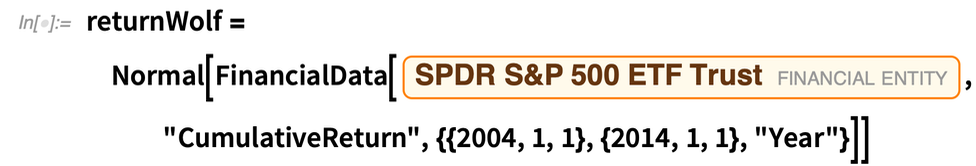✕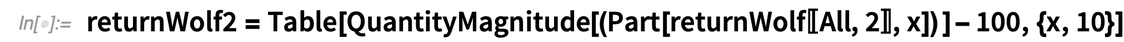✕

The next step in the assignment is to create your own functions to compute both the average and standard deviation and to sample a random value from the normal distribution defined by this average and standard deviation. When writing these self-created functions in the Wolfram Language, I discovered four best practices:

• Comments in the Wolfram Language are created using (* *) as compared to # or "" in Python. You can also highlight a section of code input and use the shortcut Alt + / (or Command + / on macOS) for commenting.
• For loops and While loops are not the best method for looping in the Wolfram Language. Instead, use Table, Map or other Wolfram functions to speed up your code.
• Never capitalize the first letter of your self-created Wolfram Language function because Wolfram-defined functions are all written using camelcase. Also, never use _ in your function names because this denotes a pattern sequence in the Wolfram Language.
• When defining multiple functions in the Wolfram Language, use a series of different iterating variables (i.e. i, j, k, l, etc.) or use the Module function. This way, if you call multiple self-created functions, the iterating variables won’t inadvertently get mixed up.

Here’s some sample code I wrote in Python as an undergrad: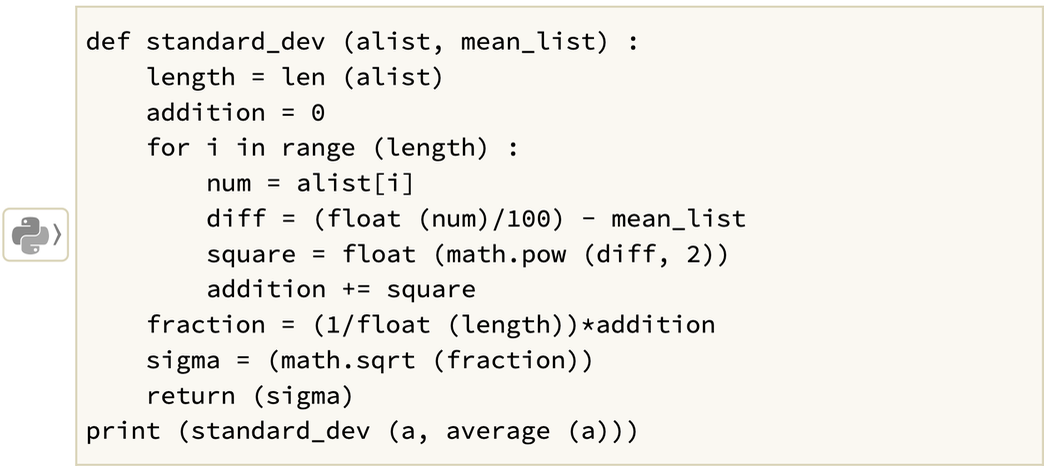✕

Here’s the comparable Wolfram Language version I wrote more recently: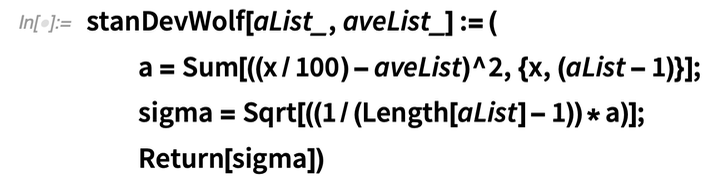✕

The next step in the assignment is to write the Monte Carlo simulation. (A complete example of the code is available in my sample Monte Carlo simulation project addendum.) When I first drafted this program in the Wolfram Language, I essentially rewrote my Python code verbatim, For loops and all. So, when I ran the Monte Carlo simulation (as advanced Wolfram Language users will understand), even a simulation of only one thousand points took demonstrably longer than I expected.

It was at this point that I realized the Wolfram Language, similar to other spoken and signed languages, has a variety of methods to structure sentences, and it requires a different structure for programming. When coming from a background with another computational language, it’s important not to assume that this language will work similarly to another language you know and are used to programming with.

When I rewrote the Wolfram Language code so it wasn’t a verbatim copy of my iterative Python code style, my Monte Carlo simulation was comparable in speed to my original Python code example.

The last steps of the assignment are easy within the Wolfram Language: creating a Histogram and obtaining the mean, standard deviation, and lower and upper 5% quantiles from my Monte Carlo simulation output. (For my undergrad assignment, all of these tasks were performed in R and Python because creating figures and performing statistics in Python was too difficult and complicated for an introductory computing course.)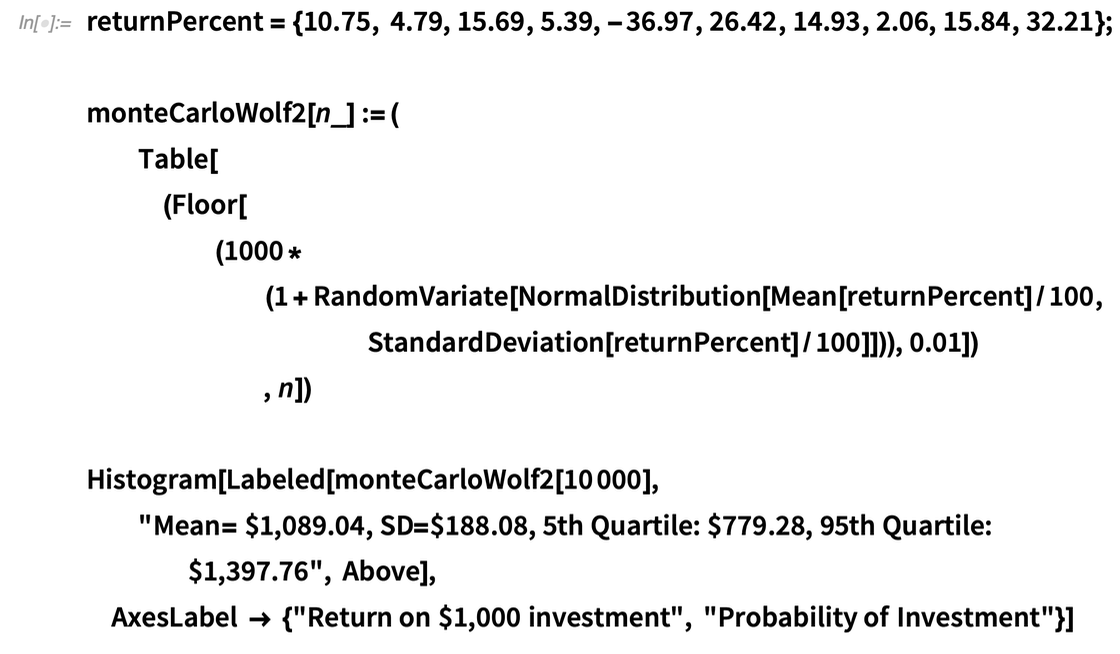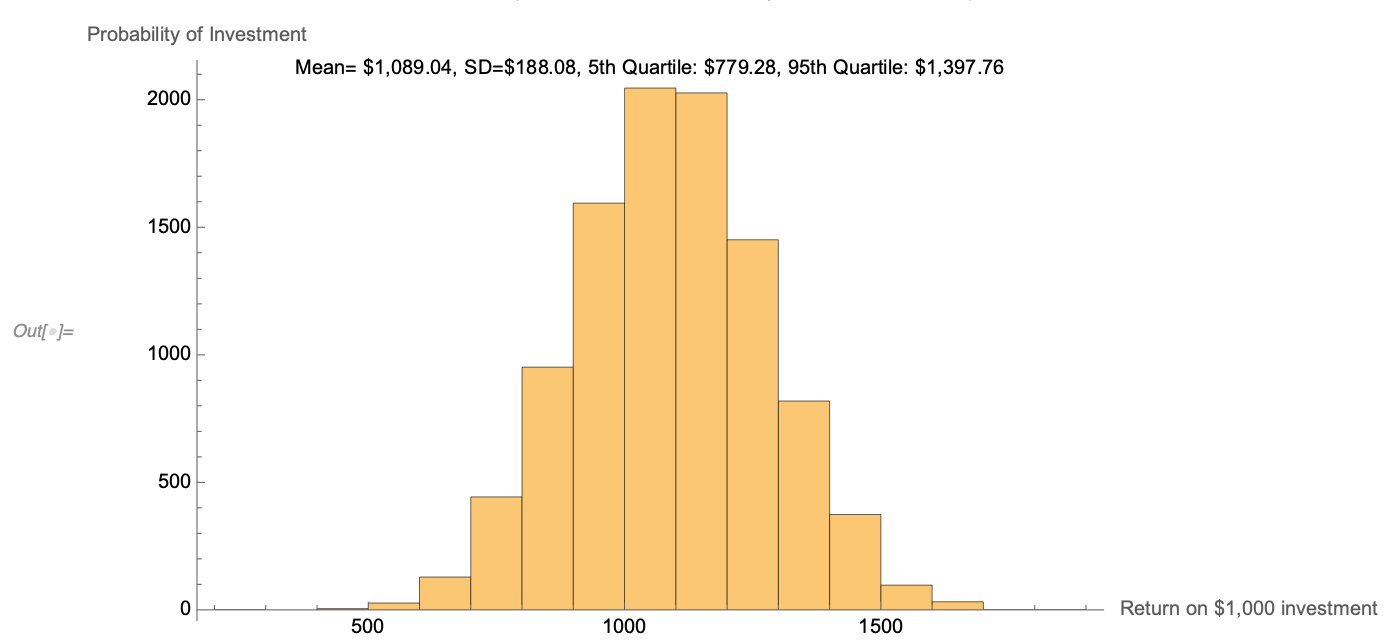✕

## Reflecting On This Experience

It was nice to program each of these Wolfram Language functions and analyze the data using a single software package. I also took the time to briefly rewrite a “discussion” on my results. Having taught multiple classes—and graded piles of homework!—as a teaching assistant throughout grad school, I have a greater appreciation for all the work faculty members do when they grade and review student homework. I recall numerous students in this undergrad computer science course forgetting to submit the discussion portion of their projects because it was a separate document than their Python and R codes.

Mathematica’s integration of both code and typesetting in the notebook interface can help prevent issues like a discussion portion not being attached with the code for a project. Moreover, a great feature that Mathematica now supports is the integration of Mathematica Online, which uses the Wolfram Cloud. Numerous universities and colleges are now supporting both Mathematica and Mathematica Online. This lets you and others access it through a web browser, phone or tablet, and also allows for a greater ease in sharing and publishing documents. I can easily see a new version of this assignment as:

“Share your completed Mathematica notebook (code, results and discussion) to *insert faculty email here* using the following naming convention: Comp_Sci_100_StudentLastName_Proj1.”

The professor’s Wolfram Cloud will send a notification when a student shares a notebook with them. The faculty member can sort the notebook into an appropriate folder and open it when they are ready to grade the assignments. I wrote an example of this in my addendum notebook at the Wolfram Notebook Archive.

## Diving In to the Timing and Speed of These Calculations

I ran multiple tests to compare the effects of different programming styles in the Wolfram Language and Python for the creation of this blog, and split the calculations into two tables. All of these calculations were performed within Mathematica, and Python calculations were performed using an external session of Python within Mathematica.

The first column shows the slow timings for what I describe as an iterative style of programming. (This style is also known as procedural programming.) The second column presents user-defined functional programming. This is when I used Table or Map as a looping function, instead of a For loop, and followed the four best practices in the Wolfram Language mentioned earlier. I did one last speed test for implementing this Monte Carlo simulation simply using built-in functions, as opposed to self-created functions. In my addendum, I also include the code for two additional Python programming styles, both of which had similar timings to this built-In function style:

Table 1: Wolfram Timings in Milliseconds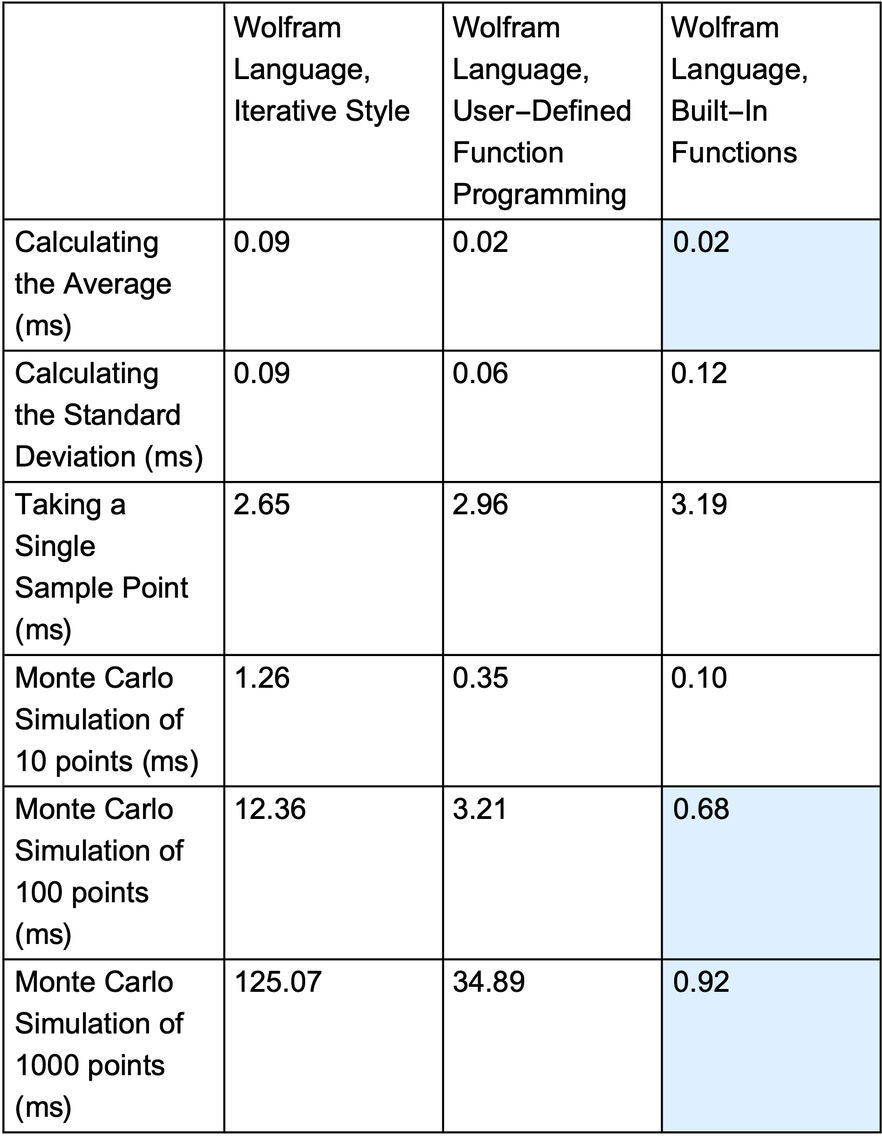✕

Table 2: Python Timings in Milliseconds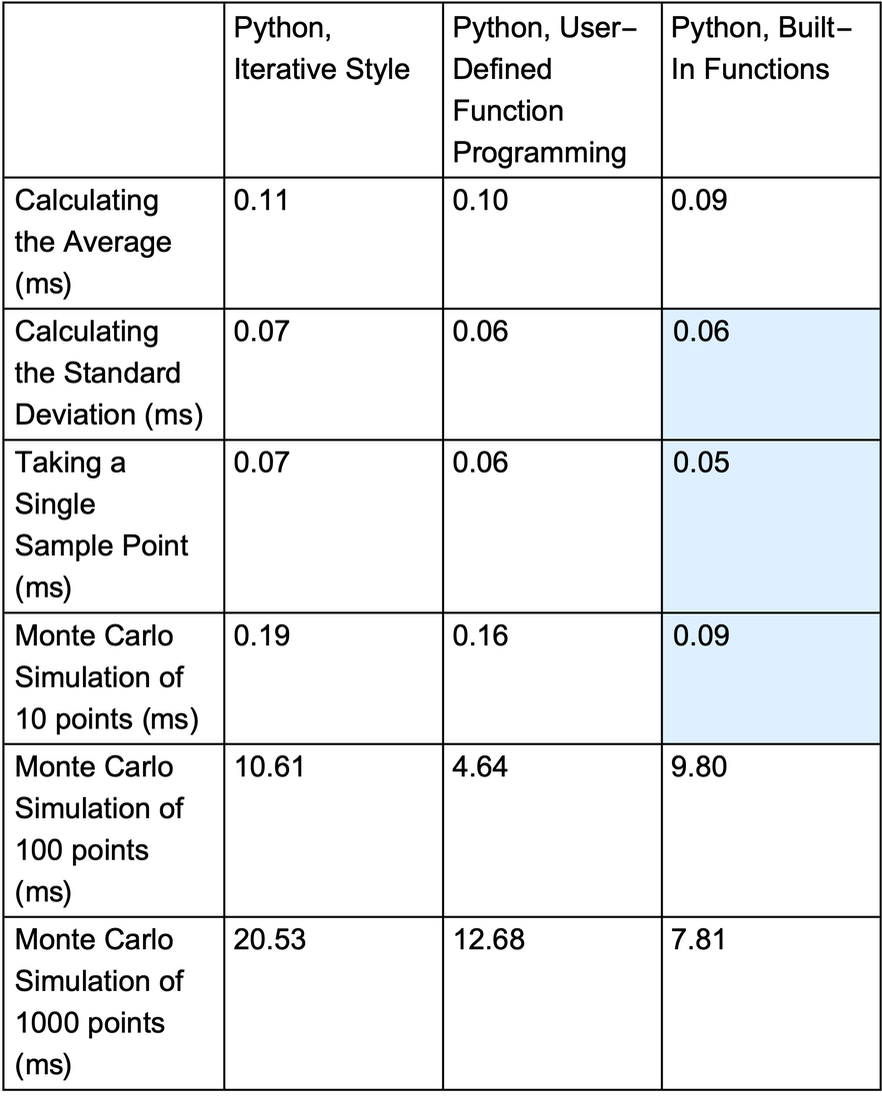✕

(A few notes: all calculations were performed in Mathematica. The average of the triplicate values is shown in each box of the respective tables. Python calculations were performed using an external session within Mathematica. A light blue background is used to denote the fastest timings. Timings were calculated using Wolfram’s AbsoluteTiming function, and a similar methodology was used with the timeit function and Version 3.9 of Python.)

As shown in the previous tables, the iterative style of programming is the slowest. Comparing the different programming styles within a single language, the Wolfram Language has a more drastic difference in speed. Although this difference in timings is apparent in the Python language, it is not nearly as dramatic. For new programmers, this initial slow speed when programming with the Wolfram Language may be a deterrent. Just as all programmers learn to write code in more efficient and effective ways, however, the substantial increases in speed associated with programming style are even more apparent in the Wolfram Language. From these tables, it’s clear that the Wolfram Language is comparable in speed to other interpreted languages like Python.

For those of us who have been classically trained in Python and other computational languages, the Wolfram Language may seem slow if we try to structure code like those other languages. If we take a step back, however, to rethink our code and the functions that we are using, the Wolfram Language has comparable speeds and a plethora of functionalities to explore. These functionalities can also make static assignments dynamic in nature to allow students to explore concepts using real-world numbers and data. Learning the Wolfram Language is just like learning any foreign language: grammar and phrasing must always be taken into account.